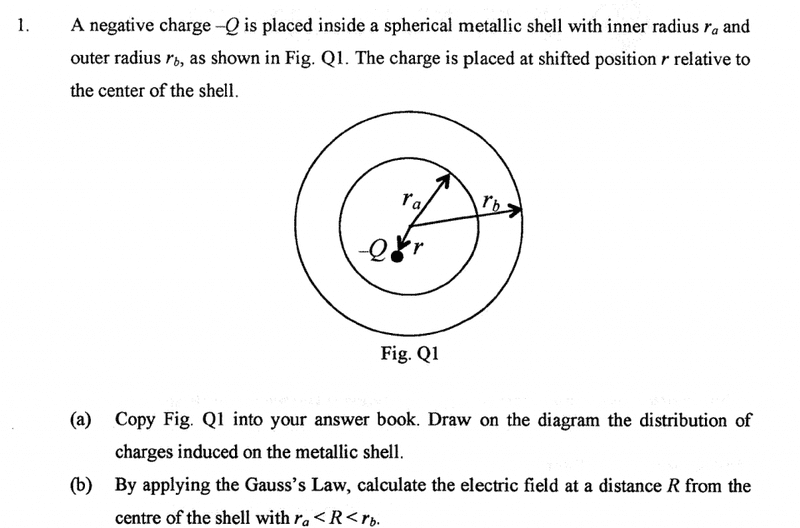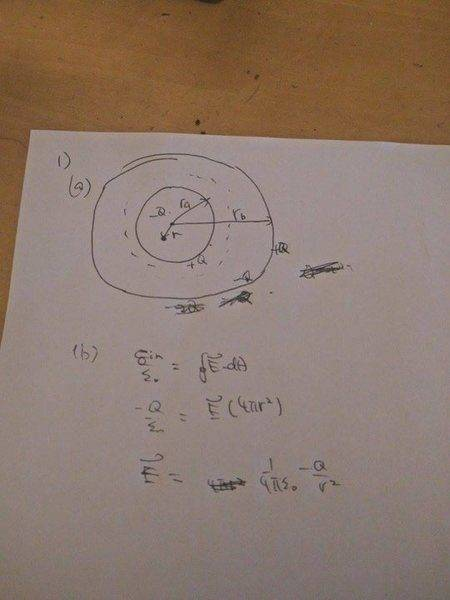# About charge inside a spherical metallic shell

• kenok1216

#### kenok1216

Poster has been reminded to use the HH Template and show their workLast edited by a moderator:

Hi kenok,Charge = 0 on the outer shell, but that doesn't mean it's zero everywhere!
What do you know about induced charges ? And about the Gauss Law ?

Last edited:
Hi kenok,Charge = 0 on the outer shell, but that doesn't mean it's zero everywhere!
What do you knwo about induced charges ? And about the Gauss Law ?
inside the met
Hi kenok,Charge = 0 on the outer shell, but that doesn't mean it's zero everywhere!
What do you knwo about induced charges ? And about the Gauss Law ?
the charge on outshell of shell1=+Q,since -Q charge inside shell1 ,net charge must be=0(since shell 1 is conductor)
then the inner shell of shell 2=-Q and outshell of shell2 =+Q?(i do not know how to explan, but it is correct?)
finally draw guess surface at ra<r<rb
qin=-Q ,so E=k-Q/r^2 when ra<r<rb

inside the met

the charge on outshell of shell1=+Q,since -Q charge inside shell1 ,net charge must be=0(since shell 1 is conductor)
then the inner shell of shell 2=-Q and outshell of shell2 =+Q?(i do not know how to explan, but it is correct)
final draw guess surface at ra<r<rb
qin=-Q ,so E=k-Q/r^2 when ra<r<rb
inside the met

the charge on outshell of shell1=+Q,since -Q charge inside shell1 ,net charge must be=0(since shell 1 is conductor)
then the inner shell of shell 2=-Q and outshell of shell2 =+Q?(i do not know how to explan, but it is correct?)
finally draw guess surface at ra<r<rb
qin=-Q ,so E=k-Q/r^2 when ra<r<rbit is correct?

(a) There is only one shell. Its inner radius is ra and its outer radius is rb.

The induced charge on the inner side of the shell is indeed +Q -- it mirrors the -Q of the charge at r.
finally draw guess surface at ra<R<rb
qin=-Q ,so E=k(-Q)/R^2 when ra<R<rb
(the symbol r is already in use for something else!)

This can't be right! You forget the -Q at r from the origin !

(a) There is only one shell. Its inner radius is ra and its outer radius is rb.

The induced charge on the inner side of the shell is indeed +Q -- it mirrors the -Q of the charge at r.
(the symbol r is already in use for something else!)

This can't be right! You forget the -Q at r from the origin !
which range of r such that E=0? i feel so confuse

No reason to feel confused ! Understanding will break through soon enough.

The -Q charge at r 'pulls' positive charge towards the inner surface of the metallic conducting shell. That positive charge will be distributed in such a way that the electric field lines inside the shell all are perpendicular to the surface. Why ? Well, if the field lines were not, the component of the electric field along the surface would cause motion of the charges until that component no longer is present.

The shell was uncharged and remains uncharged. The negative charges are repelled from the inner surface and don't hang about in the metal: they sit on the outer surface because there they have the most room (they repel each other!).

Inside a (ideal) conductor you have no electric field (reason as above: charge can move freely until there is no driving force qE left over).

And you can check this: any sphere with radius ##r_a<R<r_b## has zero charge inside, so no field !

So parts a and b of this exercise are more or less a giveaway !

No reason to feel confused ! Understanding will break through soon enough.

The -Q charge at r 'pulls' positive charge towards the inner surface of the metallic conducting shell. That positive charge will be distributed in such a way that the electric field lines inside the shell all are perpendicular to the surface. Why ? Well, if the field lines were not, the component of the electric field along the surface would cause motion of the charges until that component no longer is present.

The shell was uncharged and remains uncharged. The negative charges are repelled from the inner surface and don't hang about in the metal: they sit on the outer surface because there they have the most room (they repel each other!).

Inside a (ideal) conductor you have no electric field (reason as above: charge can move freely until there is no driving force qE left over).

And you can check this: any sphere with radius ##r_a<R<r_b## has zero charge inside, so no field !

So parts a and b of this exercise are more or less a giveaway !
so inside the shell1 q=-Q and inner shell have charge +Q , outershell have -Q
at ra<R<rb qin= -Q+Q=0 so E=0
finally i have two question below
at R<ra and R>rb E=K-Q/r^2?
between shell1 and shell2, q=0?
thanks for you help!

Last edited by a moderator:
1) at R<ra and R>rb E=K-Q/r^2?
2) between shell1 and shell2, q=+Q?
1) No and 2) No !

##R>r_b## is the easiest: ##|\vec E| = k {-Q\over R^2}## (capital R) : the charge is evenly distributed over the outer surface.
##R<r_a## is not as you describe. Check at the origin ! Is the exercise asking for this field ?

2) there is no charge between ra and rb

1) No and 2) No !

##R>r_b## is the easiest: ##|\vec E| = k {-Q\over R^2}## (capital R) : the charge is evenly distributed over the outer surface.
##R<r_a## is not as you describe. Check at the origin ! Is the exercise asking for this field ?

2) there is no charge between ra and rb
i got you point now, for R<ra just ask for the interest

For ##R<r_a## you need to find a field that has an equipotential surface at ##R=r_a##. Close to the charge -Q at r it has to be approximately like the field from a single charge.
A dipole field has such a property; the method to find it is the method of images (one of the last examples - you can swap q inside and outside)